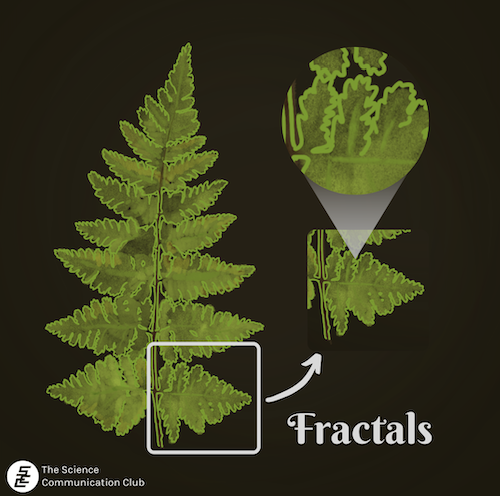# Beauty in the Eye of the Mathematician##### Written by Elakkiya Prabaharan Illustrated by Tammy Lee
What has an infinite perimeter, but a finite area?

This paradox sounds like one of those inane riddles designed to give you a headache when you’re trying to solve them, yet the answer seems so simple when you hear it. The answer to this question, however, is nothing like you’ve ever heard before. Here’s another riddle: what do ferns and the shoreline of Great Britain have in common? They are fractals!

Fractal geometry is something they probably didn’t teach you in high school math. The idea of fractal geometry, a “non-Euclidean geometry”, wasn’t solidified until the 1970s by a mathematician named Benoit Mandelbrot. We often like to think of the natural world in terms of shapes: a mountain is a triangle, the sun is a sphere, a building is a rectangular prism, and so on. But how do we define things with a more complex shape, like a cloud or the branches of a tree? Generalizing these objects into simple shapes, like circles and squares, reduces their complexity and gets rid of all the little details that make them unique. Fractal geometry seeks to correct this injustice, allowing us to describe complex, natural phenomena as mathematical shapes.

According to Mandelbrot, a fractal is “an infinitely complex pattern that is self-similar across different scales.” Self-similarity is an important feature of fractals; this essentially means that at any scale, there is a smaller part of the pattern that is like the whole. A common example of self-similarity in nature is the leaf, or frond, of a fern. The largest frond is composed of smaller fronds that are similar in shape to the large frond. When you ‘zoom’ into the smaller fronds, you’ll see that they too are composed of tinier fronds that are similar to the frond they branch from.

If you were to describe the shape of a frond using geometric terms, how might you do that? In traditional Euclidean geometry, we might describe an object by its dimension: a point has a dimension of 0, a line has a dimension of 1, a square has a dimension of 2, and a cube has a dimension of 3. Then, what is the dimension of a fractal?

Surprisingly, fractals do not have a dimension that is an integer, but rather somewhere in between. Understandably, this concept can be a little difficult to grasp—after all, we are used to describing objects as 1-, 2- or 3-dimensional. But fractal dimension measures the “roughness” of a shape, or how well it fills up space. We might intuitively think that the shape of a fern’s frond is 2-dimensional (especially when it’s laid out flat). However, it turns out that it may actually have a dimension between 1 and 2; this is because self-similar shapes often have a finite area, but an infinite perimeter. To understand this a little better, picture yourself tracing the shape of a frond on a piece of paper. How much detail would you include? Remember, each large frond is made up of smaller-sized fronds, which are each composed of even tinier fronds. Theoretically, you would be tracing the outline of the frond for a very long time in order to include every reiteration of the frond’s shape, thus the perimeter of the frond could be described as infinite. Despite this, the frond still has a finite area, which is why its dimension is neither 1 or 2 but somewhere in between. It’s a little difficult to calculate the fractal dimension of a natural fern, but British mathematician Michael Barnsey used a recursive formula to generate the image of a frond on a computer; the fractal dimension of this shape was estimated to be 1.45!

Fractals appear everywhere in the natural world. Lightning bolts, clouds, blood vessels, snowflakes, and coastlines are all fractals. These phenomena aren’t perfectly self-similar, but they contain repetitive patterns that give them fractal properties. For example, Mandelbrot famously found the fractal dimension of the coastline of Great Britain to be 1.25. At first glance, the shape of Great Britain doesn’t display self-similarity like a fern does. However, the length of its coastline appears infinite. Measuring the coastline with a ruler that is one meter long will yield a longer perimeter than if you measure it with a ruler that is one kilometer long. The more details you choose to include in your measurements (i.e. grains of sand vs. boulders of rock), the greater the perimeter. And yet, the area of Great Britain is finite.

As you can see, fractal geometry allows us to observe the natural world using mathematical terms like never before. I encourage you to look for fractals in the world around you, perhaps as you take a walk in your neighborhood; you’ll find yourself looking at nature in a whole new light!

Sources:

1. https://www.popmath.org.uk/rpamaths/rpampages/fern.html
2. https://theconversation.com/explainer-what-are-fractals-10865
3. https://users.math.yale.edu/~bbm3/web_pdfs/howLongIsTheCoastOfBritain.pdf
4. https://users.math.yale.edu/~bbm3/web_pdfs/howLongIsTheCoastOfBritain.pdf
5. https://mathigon.org/course/fractals/introduction